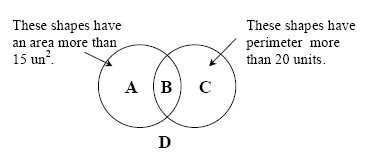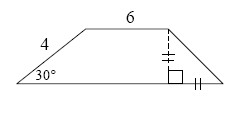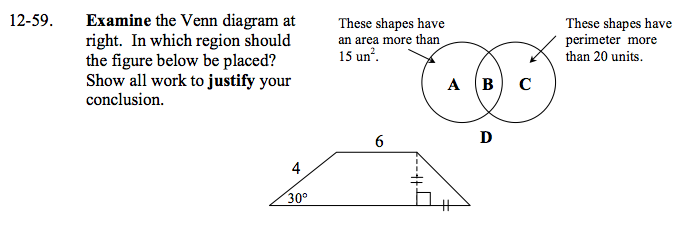### Home > GC > Chapter 12 > Lesson 12.2.1 > Problem12-59

12-59.the Venn diagram at right. In which region should the figure below be placed? Show all work to justify your conclusion. Homework Help ✎Divide the trapezoid into portions and use special right triangles to help find side lengths.
(See the online help for problem 5-122 for assistance with special right triangles).

$\text{The formula to find the area of a trapezoid is }\frac{1}{2}(\text{base}_1+\text{base}_2)(\text{height).}$

If area ≈ 17.46 units² and perimeter ≈ 24.3 units, where should the figure be placed?